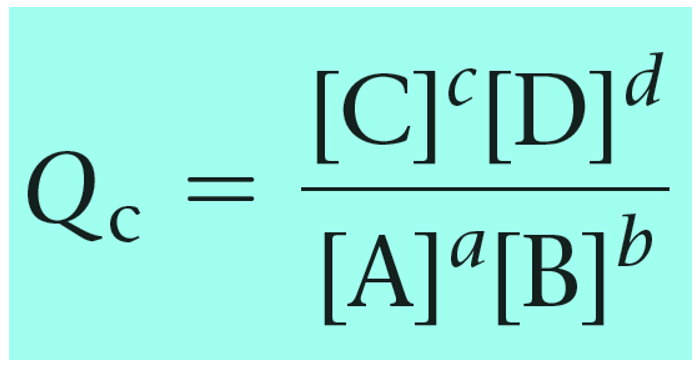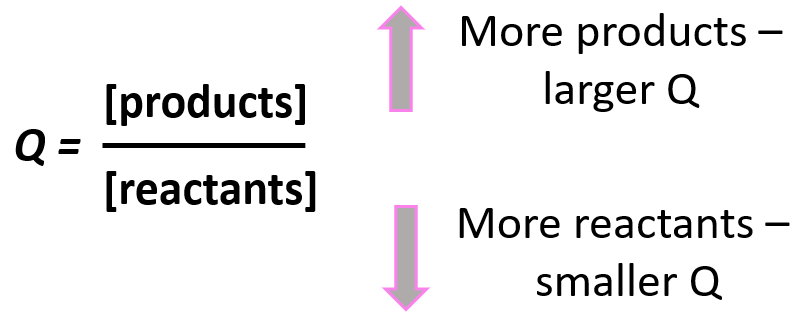## General Chemistry

To predict the direction of a reaction before an equilibrium is reached, the reaction quotient is used. It is given by the same expression as the equilibrium constant, however, instead of the equilibrium concentrations, the initial concentrations are used.

For example, the equilibrium constant Kc for the following reaction at 800 °C is 3.74 x 105

H2(g) + I2(g) ⇆ 2HI(g)

Suppose that we have a mixture of H2, I2, and HI gases at 800 K with concentrations [H2] = 0.15 M, [I2]  = 0.20 M, and [HI]  = 0.45 M.

Is the reaction mixture at equilibrium? If not, in which direction will the reaction proceed?

These are the initial concentrations of the components, and if we use them in the equilibrium constant expression, we obtain the reaction quotient Qc or Q.

${Q_{\rm{c}}}\;{\rm{ = }}\;\frac{{{{\left[ {{\rm{HI}}} \right]}^2}}}{{\left[ {{{\rm{H}}_{\rm{2}}}} \right]\left[ {{{\rm{I}}_{\rm{2}}}} \right]}}\;$

For a general chemical equation, the reaction quotient can be shown as:The reaction quotient can also be calculated based on the partial pressures of the reactant and products:Notice that the like in the expression for K, the products appear in the numerator and the reactants, in the denominator. Therefore, the more products in the reaction mixture, the larger the quotient, and vice versa – the more reactants in the mixture, the small the quotient.Going back to out problem, let’s determine the quotient and see what it tells us about the direction of the reaction:

${Q_{\rm{c}}}\;{\rm{ = }}\;\frac{{{{\left[ {{\rm{HI}}} \right]}^2}}}{{\left[ {{{\rm{H}}_{\rm{2}}}} \right]\left[ {{{\rm{I}}_{\rm{2}}}} \right]}}\; = \,\frac{{{{\left( {{\rm{0}}{\rm{.45}}} \right)}^2}}}{{{\rm{(0}}{\rm{.15)(0}}{\rm{.20)}}}}\; = \,6.75$

The quotient is smaller than the equilibrium constant (Q < K) and this means that the reaction is going to shift forward and form more of the products. The reason for this is that the reaction tends to establish equilibrium and because the equilibrium constant does not change at a given temperature (it is a constant…), the concentrators are going to change such that the quotient eventually becomes equal to the equilibrium constant.

Now, the quotient increases with the concertation of the product(s) because it appears in the numerator. Therefore, the concentration of HI is going to increase while the ones of H2 and I2 are going to decrease since they react to form more products.

If Q > K, then there is more product in the mixture than there would have been at equilibrium. Therefore, the reaction will shift to the left to decrease the concentration of the product(s) by forming more reactants.

Remember, the terms reactant and product for the equilibrium are assigned for the components on the left and the right respectively.

If Q = K, the reaction is already at equilibrium, and therefore, the concentrations are not going to change.To summarize, remember, if;

• Q < K Reaction tends to form more products.
• Q > K Reaction tends to form more reactants.
• Q = K Reaction is already at equilibrium.

The reaction quotient is used for calculating the equilibrium concentrations of the reactants and products by setting up an ICE table based on the initial, reacting, and equilibrium concentrations of the reaction components. We will cover this in the next article.

Check Also